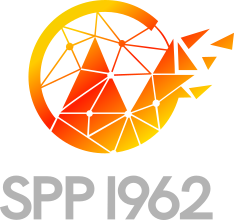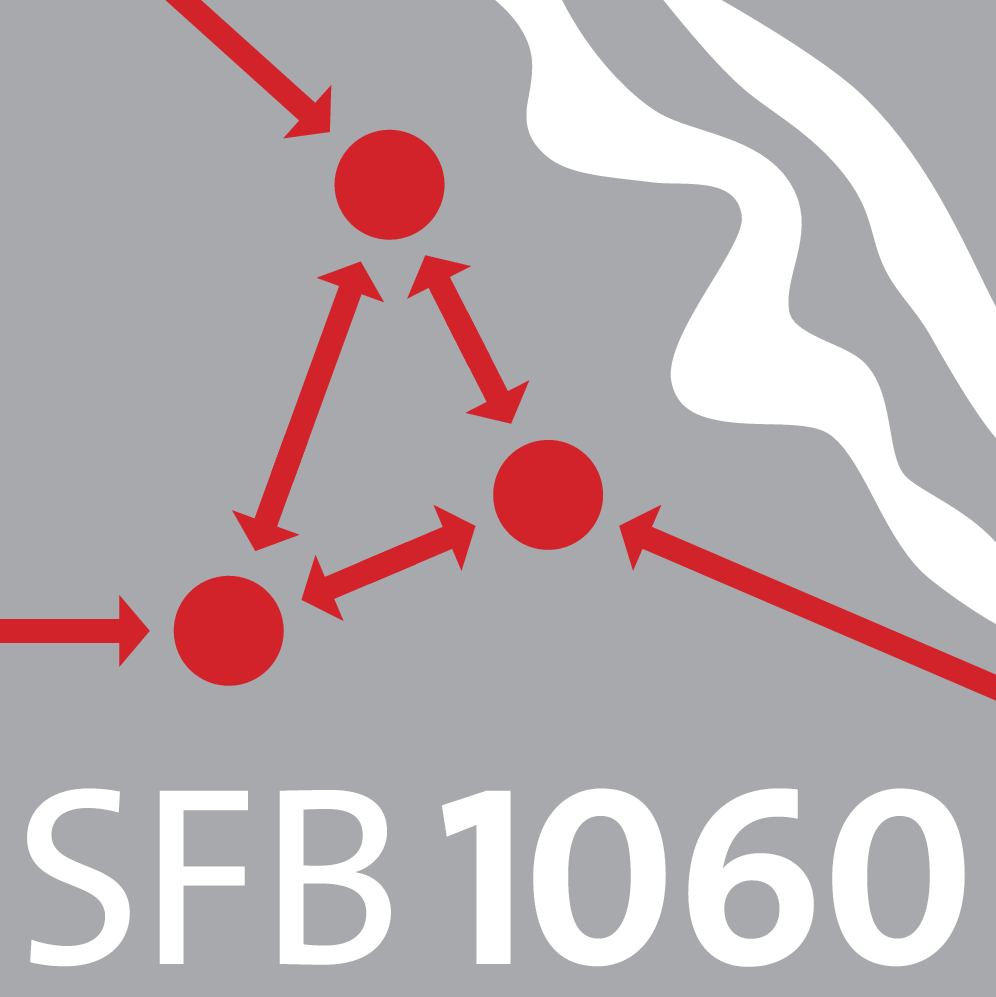# Research Group of Prof. Dr. Ira Neitzel### Contact Information

Institut für Numerische Simulation
Friedrich-Hirzebruch-Allee 7
53115 Bonn
Phone: +49 228 73-69837
Office: FHA7 3.034
E-Mail: ed tod nnob-inu tod sni ta leztiena tod b@foo tod de

### Current Research Projects### Optimizing Fracture Propagation Using a Phase-Field Approach

Project SPP 1962, DFG priority program 1962.

### Optimizing Fracture Propagation Using a Phase-Field Approach, Part II

Project SPP 1962 Phase 2, DFG priority program 1962, Phase II.### Sparse controls in optimization of quasilinear partial differential equations

Project C10, DFG SFB 1060.

### Publications

#### Submitted Articles

1. Purely time-dependent optimal control of quasilinear parabolic PDEs with sparsity enforcing penalization. F. Hoppe and I. Neitzel. Submitted. Available as INS Preprint No. 2201, 2022.
2. A note on source term representation for control-and-state-constrained parabolic control problems with purely time-dependent control. I. Neitzel. Technical Report, Institute for Numerical Simulation, Bonn University, 2022. Submitted. Available as INS Preprint No. 2206.

#### Journal Papers

1. Second Order Optimality Conditions for an Optimal Control Problem Governed by a Regularized Phase-Field Fracture Propagation Model. A. Hehl and I. Neitzel. Optimization, 2022.
2. Optimal Control of Quasilinear Parabolic PDEs with State-Constraints. F. Hoppe and I. Neitzel. SIAM J. Control Optim., 60(1):330–354, 2022.
3. Multigoal-oriented optimal control problems with nonlinear pde constraints. B. Endthmayer, U. Langer, I. Neitzel, T. Wick, and W. Wollner. Comput. Math. Appl., pages 3001–3026, 2020. Preprint available, SPP1962-108.
4. Finite element error estimates for elliptic optimal control by bv functions. D. Hafemeyer, F. Mannel, I. Neitzel, and B. Vexler. Mathematical Control & Related Fields, 10(2):333–363, 2020. Preprint version available arXiv:1902.05893.
5. Convergence of the SQP method for quasilinear parabolic optimal control problems. F. Hoppe and I. Neitzel. Optim. Eng., 2020.
6. A Lagrange multiplier method for semilinear elliptic state constrained optimal control problems. V. Karl, I. Neitzel, and D. Wachsmuth. Comput. Optim. Appl., pages 831–869, 2020. preprint available SPP1962-087.
7. First-order conditions for the optimal control of the obstacle problem with state constraints. I. Neitzel and G. Wachsmuth. PAFA, 2020. also available as arXive Preprint arXiv:2012.15324.
8. A sparse control approach to optimal sensor placement in pde-constrained parameter estimation problems. I. Neitzel, K. Pieper, B. Vexler, and D. Walter. Numer. Math., 143:943–984, 2019. also available as preprint IGDK-2018-07.
9. An optimal control problem governed by a regularized phase field fracture propagation model. Part II the regularization limit. I. Neitzel, T. Wick, and W. Wollner. SIAM J. Control Optim., 57(3):1672–1690, 2019. also available as SPP 1962 Preprint SPP1962-91.
10. Second order optimality conditions for optimal control of quasilinear parabolic pdes. L. Bonifacius and I. Neitzel. Mathematical Control and Related Fields, 8(1):1–34, 2018. Preprint version available as INS Preprint No. 1705.
11. A priori error estimates for state constrained semilinear parabolic optimal control problems. F. Ludovici, I. Neitzel, and W. Wollner. J. Optim. Theory Appl., 178(2):317–348, 2018. also available as INS Preprint No. 1605.
12. An optimal control problem governed by a regularized phase field fracture propagation model. I. Neitzel, T. Wick, and W. Wollner. SIAM J. Control Optim., 55(4):2271–2288, 2017. also available as IGDK 1754 Preprint 2015-12.
13. A priori $l^2$-discretization error estimates for the state in elliptic optimization problems with pointwise inequality state constraints. I. Neitzel and W. Wollner. Numer. Math., 2017. also available as INS Preprint No. 1606.
14. Dirichlet control of elliptic state constrained problems. M. Mateos and I. Neitzel. Comput. Optim. Appl., 2015.
15. An adaptive numerical method for semi-infinite elliptic control problems based on error estimates. P. Merino, I. Neitzel, and F. Tröltzsch. Optim. Methods Softw., 30(3):492–515, 2015.
16. Finite element discretization of state-constrained elliptic optimal control problems with semilinear state equation. I. Neitzel, J. Pfefferer, and A. Rösch. SIAM J. Control Optim., 53(2):874–904, 2015.
17. Regularization for semilinear elliptic optimal control problems with pointwise state and control constraints. K. Krumbiegel, I. Neitzel, and A. Rösch. Comput. Optim. Appl., 52(1):181–207, 2012.
18. A priori error estimates for space-time finite element discretization of semilinear parabolic optimal control problems. I. Neitzel and B. Vexler. Numer. Math., 120(2):345–386, 2012.
19. On linear-quadratic elliptic control problems of semi-infinite type. P. Merino, I. Neitzel, and F. Tröltzsch. Appl. Anal., 90(6):1047–1074, 2011.
20. A smooth regularization of the projection formula for constrained parabolic optimal control problems. I. Neitzel, U. Prüfert, and T. Slawig. Numer. Funct. Anal. Optim., 32(12):1283–1315, 2011.
21. Sufficient optimality conditions for the Moreau-Yosida-type regularization concept applied to semilinear elliptic optimal control problems with pointwise state constraints. K. Krumbiegel, I. Neitzel, and A. Rösch. Ann. Acad. Rom. Sci. Ser. Math. Appl., 2(2):222–246, 2010.
22. Error estimates for the finite element discretization of semi-infinite elliptic optimal control problems. P. Merino, I. Neitzel, and F. Tröltzsch. Discuss. Math. Differ. Incl. Control Optim., 30(2):221–236, 2010.
23. Strategies for time-dependent PDE control with inequality constraints using an integrated modeling and simulation environment. I. Neitzel, U. Prüfert, and T. Slawig. Numer. Algorithms, 50(3):241–269, 2009.
24. On regularization methods for the numerical solution of parabolic control problems with pointwise state constraints. I. Neitzel and F. Tröltzsch. ESAIM Control Optim. Calc. Var., 15(2):426–453, 2009.
25. On convergence of regularization methods for nonlinear parabolic optimal control problems with control and state constraints. I. Neitzel and F. Tröltzsch. Control Cybernet., 37(4):1013–1043, 2008.

#### Proceedings, Series- and Book Contributions

1. A-posteriori error estimation of discrete pod models for pde-constrained optimal control. M. Gubisch, I. Neitzel, and S. Volkwein. In Model reduction and approximation: theory and algorithms, volume of Computational Science and Engineering, pages. SIAM.
2. Optimizing fracture propagation using a phase-field approach. A. Hehl, M. Mohammadi, I. Neitzel, and W. Wollner. In to appear. 2020.
3. Mesh adaptivity and error estimates applied to a regularized p-laplacian constrained optimal control problem for multiple quantities of interest. B. Endtmayer, Langer, Ulrich, I. Neitzel, T. W. Wick, and W. Wollner. PAMM, 2019.
4. A priori error estimates for nonstationary optimal control problems with gradient constraints. F. Ludovici, I. Neitzel, and W. Wollner. PAMM, 15(1):611–612, 2015.
5. Numerical analysis of state-constrained optimal control problems for PDEs. I. Neitzel and F. Tröltzsch. In Constrained optimization and optimal control for partial differential equations, volume 160 of Internat. Ser. Numer. Math., pages 467–482. Birkhäuser/Springer Basel AG, Basel, 2012.
6. Optimal pde control using comsol multiphysics. I. Neitzel, U. Prüfert, and T. Slawig. Proceedings CD of the 2008 European COMSOL Conference, 2008.
7. Solving time-dependent optimal control problems in comsol multiphysics. I. Neitzel, U. Prüfert, and T. Slawig. Proceedings CD of the 2008 European COMSOL Conference, 2008.